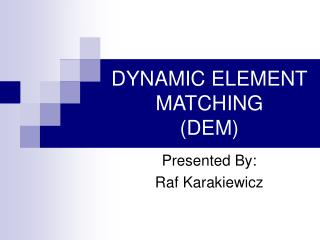# DYNAMIC ELEMENT MATCHING (DEM) - PowerPoint PPT PresentationDownload PresentationDYNAMIC ELEMENT MATCHING (DEM)

DYNAMIC ELEMENT MATCHING (DEM)
Download Presentation## DYNAMIC ELEMENT MATCHING (DEM)

- - - - - - - - - - - - - - - - - - - - - - - - - - - E N D - - - - - - - - - - - - - - - - - - - - - - - - - - -
##### Presentation Transcript

1. DYNAMIC ELEMENT MATCHING(DEM) Presented By: Raf Karakiewicz

2. Presentation Outline • With vs. Without DEM • Technical Aspects • Published Results

3. DAC (No DEM) E N C O D E R S U M 1-bit DAC 1-bit DAC 1-bit DAC 1-bit DAC 3 + 1-bit DAC X[n] 1-bit DAC - 1-bit DAC 1-bit DAC

4. DAC (No DEM) E N C O D E R S U M 1-bit DAC 1-bit DAC 1-bit DAC 1-bit DAC 3 + 1-bit DAC X[n] 1-bit DAC - 1-bit DAC 1-bit DAC

5. DAC (No DEM) E N C O D E R S U M 1-bit DAC 1-bit DAC 1-bit DAC 1-bit DAC 3 + 1-bit DAC X[n] 1-bit DAC - 1-bit DAC 1-bit DAC

6. Higher Resolution • Without DEM • Laser Trimming • Digital calibration • Careful layout • With DEM • Flawed-elements taken as they are

7. DAC (With DEM) DEM Code E N C O D E R S C R A M B L E R S U M 1-bit DAC 1-bit DAC 1-bit DAC 1-bit DAC 3 + 1-bit DAC X[n] 1-bit DAC - 1-bit DAC 1-bit DAC

8. DAC (With DEM) DEM Code E N C O D E R S C R A M B L E R S U M 1-bit DAC 1-bit DAC 1-bit DAC 1-bit DAC 3 + 1-bit DAC X[n] 1-bit DAC - 1-bit DAC 1-bit DAC

9. DAC (With DEM) DEM Code E N C O D E R S C R A M B L E R S U M 1-bit DAC 1-bit DAC 1-bit DAC 1-bit DAC 3 + 1-bit DAC X[n] 1-bit DAC - 1-bit DAC 1-bit DAC

10. DAC (With DEM) DEM Code E N C O D E R S C R A M B L E R S U M 1-bit DAC 1-bit DAC 1-bit DAC 1-bit DAC 3 + 1-bit DAC X[n] 1-bit DAC - 1-bit DAC 1-bit DAC

11. Novelty? • DEM Algorithms • How many switches? • RC ladder (L1, L2, … ) • How to switch? • Glitches • How many samples per sum? • Accuracy

12. Novelty? S C R A M B L E R S U M 1-bit DAC • DEM Algorithms • How many switches? • RC ladder (L1, L2, … ) • How to switch? • Glitches • How many samples per sum? • Accuracy 1-bit DAC 1-bit DAC 1-bit DAC + 1-bit DAC 1-bit DAC - 1-bit DAC 1-bit DAC

13. Novelty? • DEM Algorithms • How many switches? • RC ladder (L1, L2, … ) • How to switch? • Glitches • How many samples per sum? • Accuracy

14. Quantization Noise Mismatch Noise Mismatch Noise DEM L2 Mismatch Noise DEM L3

15. Noise Shaping Mismatch Noise First Order DEM Mismatch Noise Second Order DEM

16. What to take away from this presentation?

17. References •   A low-complexity dynamic element matching technique for reduced-distortion digital-to-analog conversionJensen, H.T.; Jensen, J.F.;Circuits and Systems, 1999. ISCAS '99. Proceedings of the 1999 IEEE International Symposium on , Volume: 2 , 30 May-2 June 1999 •  A performance analysis of the partial randomization dynamic element matching DAC architectureJensen, H.T.; Galton, I.;Circuits and Systems, 1997. ISCAS '97., Proceedings of 1997 IEEE International Symposium on , Volume: 1 , 9-12 June 1997 •   Dynamic element matching techniques with arbitrary noise shaping functionHenderson, R.K.; Nys, O.J.A.P.;Circuits and Systems, 1996. ISCAS '96., 'Connecting the World'., 1996 IEEE International Symposium on , Volume: 1 , 12-15 May 1996 •   Dynamic element matching in D/A converters with restricted scramblingVesterbacka, M.; Rudberg, M.; Wikner, J.J.; Andersson, N.U.;Electronics, Circuits and Systems, 2000. ICECS 2000. The 7th IEEE International Conference on , Volume: 1 , 17-20 Dec. 2000 •   An analysis of dynamic element matching techniques in sigma-delta modulationNys, O.J.A.P.; Henderson, R.K.;Circuits and Systems, 1996. ISCAS '96., 'Connecting the World'., 1996 IEEE International Symposium on , Volume: 1 , 12-15 May 1996